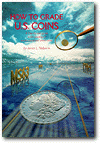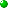IntroductionPrefaceGlossaryThe Grading ProcessHow To Grade Mint State CoinsSurface PreservationStrikeLustreEye-AppealHomeDetermining GradeIs It Proof Or Business Strike?Prooflike CoinsGrading Other SeriesWhy Won't They Grade My Coin?High End vs. Low End; The Bust-Out GameComputer GradingConclusionAbout the Author

Use the following formula, once you have assigned a numerical grade to the surface preservation, strike, lustre and eye-appeal of each side of a coin:

OBVERSE:
 Surface Preservation (1-5) ____________ x2 = ______________ Strike (1-5) = ______________ Lustre (1-5) = ______________ Eye-appeal (1-5) = ______________ OBVERSE TOTAL: ______________
REVERSE:
 Surface Preservation (1-5) ____________x2 = ______________ Strike (1-5) = ______________ Lustre (1-5) = ______________ Eye-appeal (1-5) = ______________ REVERSE TOTAL: ______________

Now we relate the following totals to corresponding grades:

 5 to 12.99 = MS or Proof-60 13 to 13.99 = MS or Proof-61 14 to 17.49 = MS or Proof-62 17.5 to 18.99 = MS or Proof-63 19 to 20.49 = MS or Proof-64 20.5 to 21.99 = MS or Proof-65 22 to 22.99 = MS or Proof-66 23 to 23.99 = MS or Proof-67 24 to 24.49 = MS or Proof-68 24.5 to 24.99 = MS or Proof-69 25 = MS or Proof-70

For example, a proof coin with an 18 obverse and a 21 reverse could be graded Proof-63/65. However, its overall grading would be Proof-63 since a coin's grade is largely determined by its worst side.

Still, usually the obverse of a coin is considered more important than the reverse. The consensus today is that the value of a coin is determined approximately 60/40 obverse to reverse. In other words, the obverse is about 11/2 times more important than the reverse. Practically the only exception occurs in the case of certain commemorative coins and patterns. In these cases, it is somewhat ambiguous which side is the obverse. Both sides are of approximately equal importance in these instances.

For this reason, it may sometimes be considered permissible to upgrade the reverse grade a bit if the obverse is toward the upper end of the scale of its grade. For example, a coin with a 20 obverse and an 18.9 reverse might still be graded MS-64. However, a coin with an 18.9 obverse and a 20 reverse (a far more common occurrence) must always be graded MS-63.